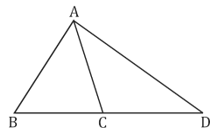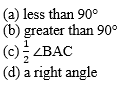Dear Students!!! There is most general as well as a scoring section in all the competitive entrance examinations in the teaching field i.e "Mathematics".Because in this section only one thing is work i.e your accuracy and that could be nourished with the daily practice. So, for this, we are providing you the daily quiz for all teaching exams i.e CTET Exam 2019DSSSB ,KVS,STET Exam.

Q1. In the given figure, if ∠B = ∠ACB = 48° and ∠D = 37°, then the value of ∠CAD is:(a) 11
(b) 10
(c) 12
(d) 13

Q2. ABC is a triangle; AD is ⊥ to BC and AE, the bisector of angle A. The measure of ∠DAE is:(a) 60
(b) 80
(c) 90
(d) 100

Q4. In a triangle ABC, AD the bisector of angle A is ⊥ to CD such that AD meets BC to D, then

(a) AB = BC
(b) AB = AC
(c) AC = BC
(d) None of these

Q5. In a parallelogram shown below, X is mid point of AD and Y is mid point of BC, XY divides DB in the ratio.(a) 1 : 2
(b) 1 : 1
(c) 1 : 3
(d) 2 : 3

Q6. On the sides of a triangle PQR, equilateral triangles SQR, TRR and UPQ are drawn externally. If ratio of sides of triangle is 2 : 3 : 4, then ratio of PU : RT : QS is:

(a) 1 : 1 : 1
(b) 2 : 3 : 5
(c) 2 : 3 : 4
(d) Can’t be determined

Q7. ABC is an equilateral triangle. If straight line ⊥ to AB, BC, CA through the points B, C, A respectively form a ∆PQR. The ratio of ∆PQR and ∆ABC is:

(a) 2 : 1
(b) 4 : 1
(c) 3 : 2
(d) 3 : 1

Q8. ABC is a right angled isosceles triangle in which AB is hypotenuse, the bisector of ∠BAC is AD meeting BC in D, then

(a) AC + CD < AB
(b) AC + CD > AB
(c) AC – CD = AB
(d) AC + CD = AB

Q9. If the bisector of angle A of triangle ABC meets BC in D, then BD : DC is equal to:

(c) AB : CD
(d) AB : AC

Q10. BO and CO are the bisectors of angles ABC and ACB of a ∆ABC, then ∠BOC is:Solutions

S1. Ans.(a)
Sol.

S2. Ans.(c)
Sol.

S3. Ans.(b)
Sol. x = 30 + 50.

S4. Ans.(b)
Sol.

S5. Ans.(b)
Sol.

S6. Ans.(c)
Sol.

S7. Ans.(d)
Sol.

S8. Ans.(d)
Sol.

S9. Ans.(d)
Sol.

S10. Ans.(b)
Sol.